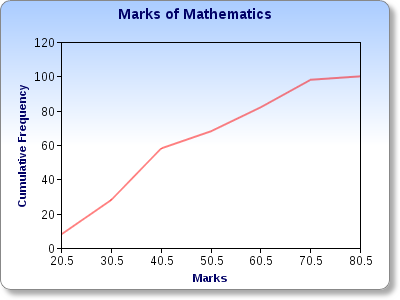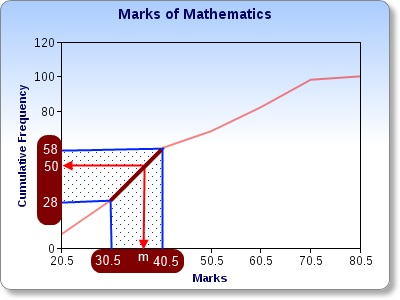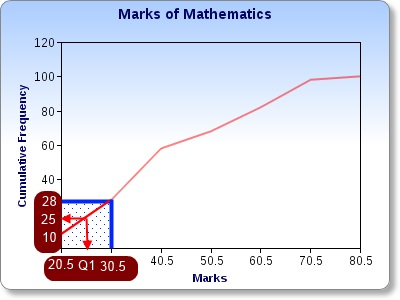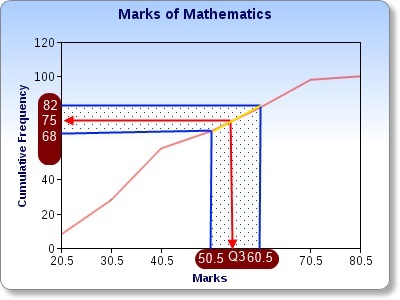# Mathematics

### Linear Interpolation

The following table shows the marks obtained for mathematics in a certain group:

ClassFrequency
11 - 2010
21 - 3018
31 - 4030
41 - 5010
51 - 6014
61 - 7016
71 - 802

Now, let's make the corresponding cumulative frequency table for the same data. It is as follows:

ClassFrequencyCumulative Frequency
11 - 201010
21 - 301828
31 - 403058
41 - 501068
51 - 601482
61 - 701698
71 - 802100

In order to plot a cumulative frequency graph, we have to plot cumulative frequency against the upper-class-boundary of each class. The curve should look like the following:#### Finding the median

The median is the n/2 th value.
100/2 = 50
50th value.

50th value lies in the 31 - 40 class - i.e. anywhere between 30.5 and 40.5. We use linear interpolation to find it.

Since we treat the segment of the curve as a straight line in this class - shown in brown colour - the process is called linear interpolation. Let's consider the gradient of the line segment as follows:So, 30/10 = 22/(m-30.5)
3 = 22/(m-30.5)
3m - 91.5 = 22
3m = 113.5
m = 37.8
median = 37.8

#### Finding the first quartile - Q1

The first quartile is the n/4 th value.
100/4 = 25
25th value.

25th value lies in the 21 - 30 class - i.e. anywhere between 20.5 and 30.5. We use linear interpolation to find it.

Let's consider the gradient of the red line segment as follows:So, 18/10 = 15/(Q1-20.5)
1.8 = 15/(Q1-20.5)
1.8Q1 - 36.9 = 15
1.8Q1 = 51.9
Q1 = 28.8
First Quartile = 28.8

#### Finding the third quartile - Q3

The third quartile is the 3n/4 th value.
100 X (3/4) = 75
75th value.

75th value lies in the 51 - 60 class - i.e. anywhere between 50.5 and 60.5. We use linear interpolation to find it.

Let's consider the gradient of the yellow line segment as follows:So, 14/10 = 7/(Q3-50.5)
1.4 = 7/(Q3-50.5)
1.4Q3 - 70.7 = 7
1.4Q3 = 77.7
Q3 = 55.5
Third Quartile = 55.5Maths is challenging; so is finding the right book. K A Stroud, in this book, cleverly managed to make all the major topics crystal clear with plenty of examples; popularity of the book speak for itself - 7th edition in print.

### Recommended - GCSE & iGCSEThis is the best book available for the new GCSE(9-1) specification and iGCSE: there are plenty of worked examples; a really good collection of problems for practising; every single topic is adequately covered; the topics are organized in a logical order.

### Recommended for A LevelThis is the best book that can be recommended for the new A Level - Edexcel board: it covers every single topic in detail;lots of worked examples; ample problems for practising; beautifully and clearly presented.